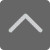## 爱智康资讯站

### 课程咨询: 4000-121-121

• 全国课程在线咨询
• 咨询热线：4000-121-121
• 登录手机官网

• 关注微信公众号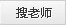# 常用的“历史万能公式”，不会也能蒙对

2019-11-28 14:53:50 　来源：西安爱智康 文章作者：西安爱智康

## 了解爱智康期末短期课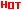* 爱智康会在1个工作日内与您取得联系

― ― 报名课程可获得 ― ―

★历史背景=（国内+国际）（经济+政治+文化+……）

（1）经济背景=生产力+生产关系+经济结构+经济格局+……

（2）政治背景=政局+制度+体制+政策+阶级+民族+外交+军事+……

（3）文化背景=思想、宗教+科技+教育+……

★原因：（1）原因广度：原因=主观（内因）+客观（外因）

（2）原因深度：→直接→主要→根本

★矛盾分析：生产力与生产关系矛盾、经济基础与上层建筑矛盾、阶级矛盾、阶级内部矛盾、民族矛盾、宗教矛盾、不同利益集团矛盾……

★目的、动机：→直接→主要→根本

⑴直接动机：解决当前面临的种种危机或问题

⑵主要目的：实现某一方面目标，求得稳定和发展等

⑶根本目的：建立或巩固统治，维护统治阶级利益（根本利益）

★经济内容：生产力+生产关系+经济结构、布局+……

①经济政策=总政策+土地+租税+赋役+人身+……

②农业经济=人口+土地+工具+水利+作物+布局+……

③手工业经济=（纺织+矿冶+陶瓷+……）（技术+布局 +……）

④商品经济=城市+交通+商品+市场+货币+边贸+外贸+……

⑤经济结构、布局=经济成份比例变化+经济重心变化+经济方式扩展+……

⑵近现代经济=经济发展要素+经济成份+国际经济+……

①经济发展要素=技术+资金+市场+原料+劳力+经济结构+经营方式+政策+……

②经济成份=自然经济+外资+合资+民资+官资+国营+集体+个体+……

③国际经济=总格局+（生产+管理+资本+技术+市场+关税）（协作+竞争）

★政治内容=制度+体制+政策+阶级+民族+外交+军事+……

⑴古代政治=政局+制度+中央+地方+阶级+军事+财政+民族+对外+……

⑵近现代政治=政局+制度+政体+体制+政权+政治力量+权力+权利+……

⑶国际政治=体系+格局+集团+合作+战争+对抗+妥协+……

★文化内容=自然科学+社会科学+文化交流+……

⑴自然科学=（科学理论+科技发明）（天文+地理+数学+物理+化学+生物+医学+农学+……）

⑵社会科学=思想、宗教+教育+史学+文学+艺术+……

⑶文化交流=（民族+对外）（传播+吸收）+文化地位+……

★事件、事态过程：准备→开始→发展或曲折→成功或失败

★性质分析：任务+领导阶级+主力+手段+结果……

★影响或意义=（国内+国际）（经济+政治+文化）+深远影响……

⑴经济影响=生产力+生产关系+经济结构+经济格局+……

⑵政治影响=格局+制度+体制+政权+政策+阶级+民族+……

⑶文化影响=思想+科技+教育+文学艺术+……

⑵成败原因=（主观+客观）（经济+政治+军事+策略+……）

⑶成败根源=（社会条件+阶级本质）（进步/局限）

★经验教训或启示：（经验+教训）→启示 ：

⑴政治=国情+领导+群众+武装+民主+法制+思想+策略+……

⑵经济=生产力+生产关系+客观规律+发展战略+……

⑶文化=批判+改造+继承+发展+……

★历史评价=(积极因素+消极因素)史实+结论

（1）人物评价=属性+事迹+影响（进步+局限）+结论

（2）事件、事态评价=概况+性质特点+影响（进步/反动+正义/非义）+结论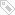标签：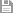保存　｜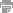打印　｜关闭